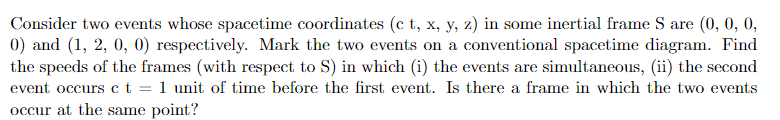# Calculating Spacetime Intervals for Simultaneous Events

• milkism

#### milkism

Homework Statement
Analyse of two events with the use of invariant intervals
Relevant Equations
I= -c²t² + d²
Exercise:My solutions:
1. For events to be simultaneous, the invariant interval must be bigger than zero (spacelike). I got $$I = -c^2 \Delta t^2 + \Delta x^2 + \Delta y^2 + \Delta z^2 = -(0-1)^2 + (0-2)^2 + (0-0)^2 + (0-0)^2 = -1 + 4 = 3 >0$$. Which is indeed greater than zero, to find the velocity, I will use the first Lorentz-transformation formula with four vectors $$\overline{x}^0 = \gamma \left( x^0 - \beta x^1 \right) = \Delta (c\overline{t}) = \gamma (\Delta (ct) - \beta (\Delta x))$$, we want $$\Delta \overline{t} = 0$$. We get: $$\Delta (ct) = \beta (\Delta x) = \frac{0-1}{0-2} = \frac{v}{c} = v = \frac{c}{2}$$. Where $$\beta = \frac{v}{c}$$.
2. The new spacetime coordinates for second event will be (-1,2,0,0), the invariant interval doesn't change, meaning it's still spacelike, so the velocity will be the same but opposite sign.
3. No, for two events to occur at the same point (place) the invariant interval must be negative (timelike), which isn't.

We get: $$\Delta (ct) = \beta (\Delta x) = \frac{0-1}{0-2} = \frac{v}{c} = v = \frac{c}{2}$$. Where $$\beta = \frac{v}{c}$$.
So you're claiming ##\Delta (ct) = \frac{c}{2}## so ##-1 = 1.5\times 10^8~\rm m/s##? Don't use an equal sign to connect steps.

The new spacetime coordinates for second event will be (-1,2,0,0), the invariant interval doesn't change, meaning it's still spacelike, so the velocity will be the same but opposite sign.
You may want to rethink this one.

•milkism, topsquark, MatinSAR and 1 other person
So you're claiming ##\Delta (ct) = \frac{c}{2}## so ##-1 = 1.5\times 10^8~\rm m/s##? Don't use an equal sign to connect steps.

You may want to rethink this one.
Are the spacetime coordinates wrong?

Are the spacetime coordinates wrong?
##v = \frac c 2## looks right for part (i), but your work seems very muddled to me. For example:

We get: $$\Delta (ct) = \beta (\Delta x) = \frac{0-1}{0-2} = \frac{v}{c} = v = \frac{c}{2}$$.
For part (ii) you need to work things out properly. You've effectively just guessed a (wrong) answer.

•milkism, topsquark and MatinSAR
##v = \frac c 2## looks right for part (i), but your work seems very muddled to me. For example:

For part (ii) you need to work things out properly. You've effectively just guessed a (wrong) answer.
Well, I'm lost.

You know the coordinates in ##S##. Try using your answer ##\beta = -1/2## and the Lorentz transformation and see what you get for the coordinates in the other frame.

•milkism and topsquark
Well, I'm lost.
This problem, IMO, is simply asking you to solve an equation for ##v##. Namely:$$\gamma(1 - 2\frac v c)= -1$$

•milkism
Haha, I thought we had to do something with invariant intervals for (ii), thanks! Is my solution for (iii) correct?

Is my solution for (iii) correct?
Yes. Note that you can prove it in this case by showing that no allowable ##v## would solve the relevant equation.

•milkism
Yes. Note that you can prove it in this case by showing that no allowable ##v## would solve the relevant equation.
Because it would be bigger than the speed of light?

Because it would be bigger than the speed of light?
I don't know. Try it!

•milkism